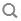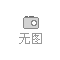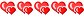点击下图片即可查看大图
•••# 意大利家具 法式家具 古典家具 欧式家具 欧式实木雕花宫廷奢华家具 法式浪漫古典卧室家具双抽彩绘床头柜99床头柜手机扫描查看

• 面议
• 起订量：77 件
• 可售数量: 77 件
• 支持批量采购
• 发布时间：2021-01-17 01:40

资料信息

• 店铺等级：• 旺铺店长: fpfurniture
• 服务保障：暂未签署消保协议
• 什么是消费者保障协议
• 认证类型：
• 身份认证：
• 状态：[离线]  [加为商友]   [发送信件]

认证信息

• 实名认证
• 信用认证
• 商品合格
• 产品详情
• 联系方式
• 产品评价
• 供货总量：77 件
• 发货期限：自买家付款之日起 天内发货
• 所在地：

rr

r r r r r r r r r r r r r r r r r r r r r r r r r r r r r r r r r r r r r r r r r r r
 r 包装体积:0.5r r 是否可预售:是r r 是否组装:整装r r 门数量:无门r r 高度:600mm(含)-850mm(不含)r r 是否带滚轮:否r r 抽屉导轨节数:3节r r 是否带阻尼:不带阻尼r r 风格:欧式r r 图案:艺术r r 附加功能:储藏r r 家具结构:框架结构r r 产地:广东省r r 城市:佛山市r r 材质:木r r 木质材质:橡胶木r r 是否可定制:是r r 饰面材质:木r r 适用对象:成人r r 可送货/安装:r
rr rr

rr rr

rr rr

rr rr

rr rr

r r r r r r r r r r r r r r r r r r r r r r r r r r r r r r r r r r r r r r r r r r r r r r r r r r r r r r
 r 【名称】r r FP菲帕家居 田园 欧式 实木家具 法式浪漫 卧室 双抽床头柜r r 【风格】r r  欧式r r 【品牌】r r  菲帕家居（Filiphs Palladio）r r 【公司】r r  佛山市南海沛俪菲帕家具厂r r  【颜色】r r  裂纹漆颜色r r 【同类推荐】r r  查看同系列配套产品 r r 【包装运输】r r  新型3CM厚蜂窝纸板包装r r 【产地】r r  广东佛山r r 【生产周期】r r  30-45天左右可发货 3-10天左右的运输期r r 【保养】r r  无须保养r r 【运费】r r  运费都是到付的 一般需要顾客到当地市区物流点自提货物r r 【产品尺寸】r r r 长*宽*高:0.55*0.45*0.61m r r r 【材料】r r r  1.全实木框架(包括雕花部分)+胶合板+环保底漆+环保裂纹漆+台湾金箔,r 2.精选五金配件，经多次开合实验合格，回弹力度均匀;（传统风格的古典家具采用实木导轨，如喜欢推拉更顺畅的金属导轨，请与客服沟通）r r r 【包装箱数】r r  1件r r 【包装细节】r r  1.**层：产品;r 2.第二层：气泡膜+珍珠棉;r 3.第三层：3CM厚峰窝纸板搭建的板箱+护角纸r
rr r 长*宽*高:0.55*0.45*0.61m r

rr rr

rr rr

rr rr

rr rr

rr rr

r• 产品详情
• 联系方式
• 产品评价